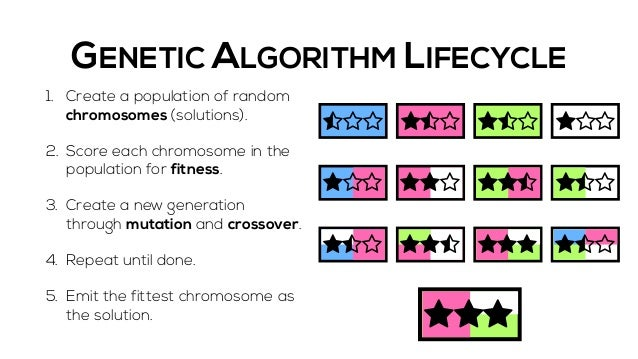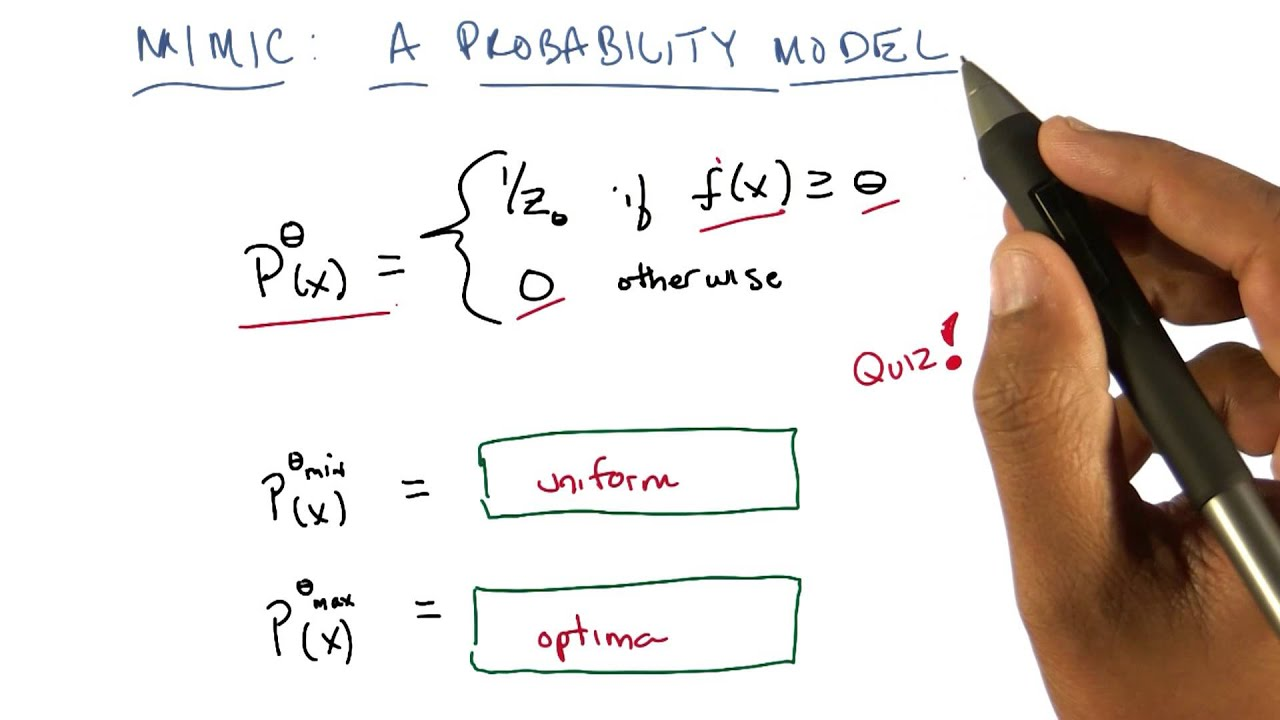# Unsupervised Learning: Randomized Optimization

This is the 12th in a series of class notes as I go through the Georgia Tech/Udacity Machine Learning course. The class textbook is Machine Learning by Tom Mitchell.

This marks the start of a new miniseries on Unsupervised Learning, the 2nd of 3 sub disciplines within Machine Learning.

## Our Goal: Optimization🔗

Given an input space `X`, and an objective (aka fitness) function `f(x)`, Find `x` from `X` such that `f(x)` is the maximum possible it can be.

This is similar to the argmax function we have used in prior formula, but now we are actually exploring methods to do it.

Given a small `X`, we can simply run a `for` loop like this one and keep track of the highest values.

Given an infinite `X` but with a solvable `df(x)`, we can use calculus to help us find optima.

If `df(x)` can't be solved, we can use the Newton Raphson method to iteratively get closer and closer to the optimum. However, it can get stuck in local optimum.

But what happens when we don't have a derivative to work with? (for example, you don't even know what f(x) is, you just know the output without knowing its theoretical maximum) and possibly many local optima?

## Method 1: Random Restart Hill Climbing🔗

The simple solution is, to guess a few starting points and then guess a direction and try to do some random restart hill climbing. However, to do it right, you'd have to do a number of restarts and it turns out to be not a lot better than just looping through the entire space especially for spaces with narrow global optima basins.

Can we do better than that?

## Method 2: Simulated Annealing🔗

Random Restart Hill Climbing combines exploring (random restart) with exploiting (climbing). Maybe we can split that up.

Simulated Annealing takes its name from metallurgy where repeatedly heating up and cooling down a sword makes it stronger than before.The pseudocode for this looks like:

``````Start with a temperature T
For a finite set of iterations:

- sample new point x+
- jump to a new point with probability given by an acceptance probability function P(x, x+, T)
- decrease temperature T

where

P(x, x+, T) = 1 if f(x+) >= f(x) else e^((f(x+) - f(x))/T)
``````

So a high T makes the algorithm behave like a random sampler, and as it cools, it starts to behave more like a hill climber.A remarkable analytical result about SA is that the probability of it ending at X is equal to `e ^ (f(x) / T) / Z(T)` (a Boltzmann distribution) which makes the likelihood of its ending point directly related to its ending point's fitness.

## Method 3: Genetic Algorithms🔗

Evolution is a pretty good optimizer, and we can simulate that by "breeding" individual points to produce hopefully better offspring and "mutating" them by doing local search. Over multiple "generations", the hope is that we evolve towards the globally optimum result. One feature that helps a lot is if the individual dimensions/attributes can be additively combined, so that breeding two parents can regularly produce better offspring.To contrast this with the other methods, GAs are like random restarts done in parallel (since each individual in a population is like a thread, and each generation is like a restart), EXCEPT that the "crossover" of information when "breeding" happens helps to direct the general direction of evolution towards better places.

The pseudo-code looks like:

``````Start with an initial population of size K
Repeat until converge:
- compute fitness of all population
- select "most fit" individuals
- pair up individuals, replacing "least fit" via crossover/mutation
``````

As you might imagine, the secret sauce is in the breeding. Two methods of doing preserving individuals are:

• truncation selection - for example, only keeping the top half of the population
• roulette wheel selection - taking a probabilistic approach to keeping each individual according to their fitness

Crossover/Mutation also has nuances. Deciding on what attributes can be treated as a group involves domain knowledge and implicit assumptions that may or may not reflect reality.

## More Randomized Optimization🔗

As you can see, there are many ways to tackle the problem of optimization without calculus, but all of them involve some sort of random sampling and search.

Those we have explored don't have much in the way of memory or of actually learning the structure or distribution of the function space, but there are yet more algorithms that explore those abilities:

## MIMIC🔗

MIMIC has a simple idea at its core - define a uniform probability distribution of points with a fitness over a threshold `theta`:When theta is at a minimum, that means we uniformly sample over the entire space.

When theta is at a maximum, that means we converge on one or more global optima.

``````at every iteration:

- Generate samples from population consistent with the distribution so far
- set `theta` to the nth percentile
- retain only those samples where f(x) > `theta`
- estimate the new distribution based on these samples,
- repeat
``````

Therefore, under MIMIC, our task is to continually raise `theta` until we just have small regions around our optima left. In the process of doing so, we infer a (uniform) probability distribution around our optima. Because we use the `nth` best percentile as our cutoff factor, this is similar to genetic algorithms, however there is no longer a concept of breeding or genes. Instead, we stay laser focused on performance against the fitness function as our one metric.

The comparison with genetic algorithms isn't accidental - the best version of MIMIC estimates distributions by assuming that the conditional distributions inherent in the space fit to "dependency trees", which are Bayesian Networks where every node (but one) has exactly one parent. Finding these trees is out of scope but check the videos for the math, but the theoretical grounding of this approach is rooted in maximizing Mutual Information (in information theory terms) and building a Maximum Spanning Tree. While not the only way to estimate the next probability distribution, Dependency Trees allow capturing of parent-child structure without having exponential cost (Max Spanning Trees are only polynomial in cost).

Benefits:

• The fact that MIMIC's "rising theta" approach can be used with any underlying probability distribution is pretty cool - here's a simple applied example.
• Being able to learn structure via the distribution assumption helps with generalizability
• Empirical tests show MIMIC using 2-3 orders of magnitude less iterations to converge compared to Simulated Annealing and other alternatives, however, this is offset by each iteration taking much longer because of the need to estimate and generate from probability distributions. Thus, in terms of overall time taken, MIMIC works better when cost of evaluating `f(x)` is high (e.g. rocket simulation, antennae design, human test subjects)

## Next in our series🔗

Further notes on this topic:

Hopefully that was a good introduction to Randomized Optimization. I am planning more primers and would love your feedback and questions on:

• Overview
• Supervised Learning
• Unsupervised Learning
• Reinforcement Learning
• Markov Decision Processes - week of Mar 25
• "True" RL - week of Apr 1
• Game Theory - week of Apr 15